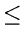Next:10.3.5 Job Shop SchedulingUp:10.3 Examples of some Intractable ProblemsPrevious:10.3.3 Knapsack Problem

## 10.3.4 Bin Packing

Suppose we have unlimited number of bins each of unit capacity and n objects with sizes s1, s2,..., sn where the sizes si(i = 1,..., n) are rational numbers in the range 0 < si1.

Optimization Problem: Determine the smallest number of bins into which the objects can be packed and find an optimal packing.

Decision Problem: Given an integer k, do the objects fit in k bins?

eEL,CSA_Dept,IISc,Bangalore